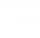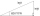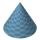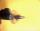# Sine + cosine - math problems

#### Number of problems found: 88

• Angles by cosine lawCalculate the size of the angles of the triangle ABC, if it is given by: a = 3 cm; b = 5 cm; c = 7 cm (use the sine and cosine theorem).
• Right triangle trigonometricsCalculate the size of the remaining sides and angles of a right triangle ABC if it is given: b = 10 cm; c = 20 cm; angle alpha = 60° and the angle beta = 30° (use the Pythagorean theorem and functions sine, cosine, tangent, cotangent)
• Q-ExamIf tg α = 9.6, Calculating sin α, cos α, cotg α .
• CotangentIf the angle α is acute, and cotg α = 1/3. Determine the value of sin α, cos α, tg α.
• One sideOne side is 36 long with a 15° incline. What is the height at the end of that side?
• The coneThe lateral surface area of the cone is 4 cm2, the area of the base of the cone is 2 cm2. Determine the angle in degrees (deviation) of the cone sine and the cone base plane. (Cone side is the segment joining the vertex cone with any point of the base c
• Trigonometric functionsIn the right triangle is: ? Find the value of s and c: ? ?
• PentagonCalculate the area of a regular pentagon, which diagonal is u=16.
• RightDetermine angles of the right triangle with the hypotenuse c and legs a, b, if: ?
• ABCDAC= 40cm , angle DAB=38 , angle DCB=58 , angle DBC=90 , DB is perpendicular on AC , find BD and AD
• TrigonometryIf you know that cos(γ) = sin (806°), what is the angle γ?
• Right triangleA right triangle ABC is given, c is a hypotenuse. Find the length of the sides a, b, the angle beta if c = 5 and angle alfa = A = 35 degrees.The angle of a straight road is approximately 12 degrees. Determine the percentage of this road.
• Chord - TSThe radius of circle k measures 68 cm. Arc GH = 47 cm. What is TS?
• SteepleSteeple seen from the road at an angle of 75°. When we zoom out to 25 meters, it is seen at an angle of 20°. What is high?
• Isosceles triangle 10In an isosceles triangle, the equal sides are 2/3 of the length of the base. Determine the measure of the base angles.
• The aspect ratioThe aspect ratio of the rectangular triangle is 13: 12: 5. Calculate the internal angles of the triangle.
• BallThe Ball was fired at an angle of 35° at an initial velocity of 292 m/s. Determine the length of the litter. (g = 9.81 m/s2).
• Regular 5-gonCalculate the area of the regular pentagon with side 7 cm.
• TriangleCalculate the area of ​​the triangle ABC if b = c = 17 cm, R = 19 cm (R is the circumradius).

Do you have an interesting mathematical word problem that you can't solve it? Submit a math problem, and we can try to solve it.

We will send a solution to your e-mail address. Solved examples are also published here. Please enter the e-mail correctly and check whether you don't have a full mailbox.

Please do not submit problems from current active competitions such as Mathematical Olympiad, correspondence seminars etc...

Most natural application of trigonometry and trigonometric functions is a calculation of the triangles. Common and less common calculations of different types of triangles offers our triangle calculator. Word trigonometry comes from Greek and literally means triangle calculation. Sine - math word problems. Cosine - math word problems.